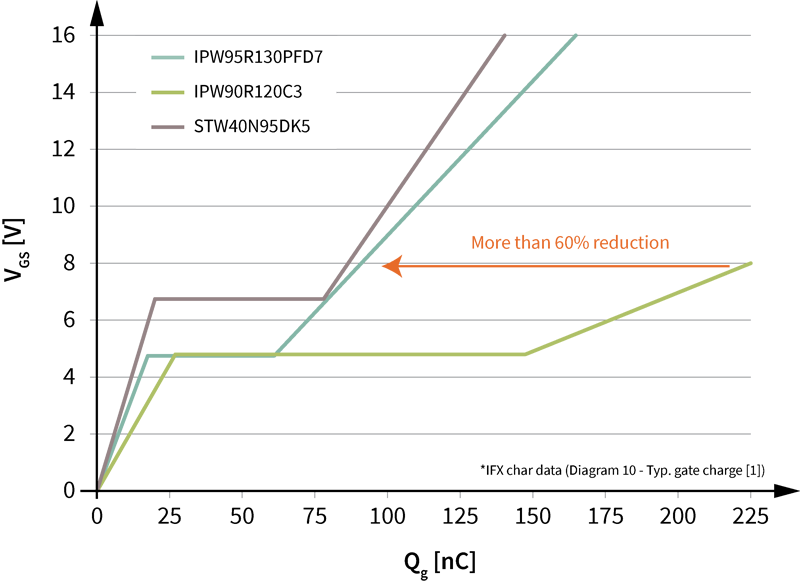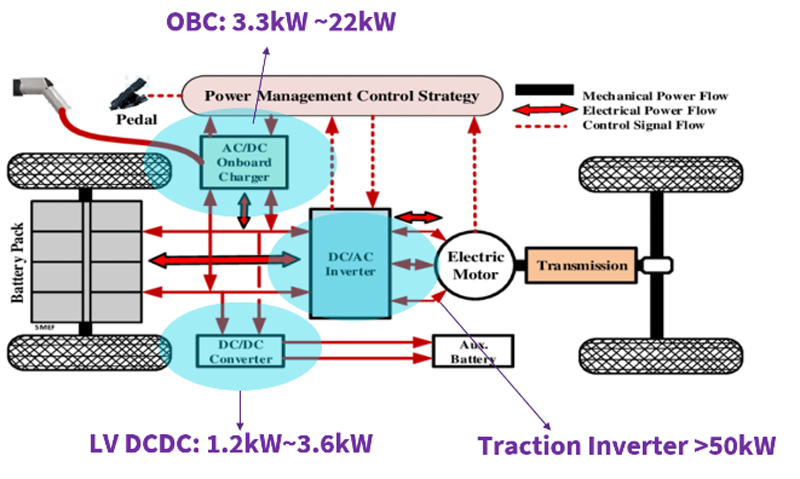# IGBTs impact efficiency and ruggedness in solar inverter apps

Author:
Satyavrat Laud, Renesas

Date
02/04/2014PDF
##### Efficiency directly relates to income generation, so device performance is paramount

In terms of performance requirements for a power device, a solar inverter is one of the most demanding industrial applications. First, for a grid tie inverter, efficiency directly relates to income generation, so device performance is paramount. Also a long and reliable life, on the order of 20 years or more, for the inverter is crucial. Meeting these requirements is a task for which the latest generation of IGBTs is ideally suited.

Typical Solar Inverter: Figure 1 shows the basic topology of a single-phase H bridge inverter (a three-phase output inverter simply adds another half bridge leg to this topology). This is a common and representative topology of most solar inverters with single phase, 60 Hz, 208 V or 240 V (RMS) voltage output in the 1 to 5 kW power output range.

Click image to enlarge

Figure 1: Typical single-phase H bridge topology solar inverter

In this topology, the power devices (i.e. IGBTs co-packed with an antiparallel Fast Recovery Diode) are typically pulse width modulated at a frequency in the 20 to 40 kHz range, resulting in conduction and switching losses in each device which have to be minimized. Further, a long inverter life demands device ruggedness and operating temperatures be considered. For this, IGBT ruggedness defined by the length of time it can withstand a short circuit across its collector and emitter terminals, and IGBT operating junction temperatures are the key factors (the cooler the device runs the longer its operating life).

As an example, an H bridge topology solar inverter with a 240Vac, 5kW, 60Hz, single-phase output is considered. A target efficiency of ≥95% at rated power output is set as a requirement, and the implications for the IGBTs in terms of loss and ruggedness are analyzed.

Loss Analysis

For a goal of ≥95% inverter efficiency at rated power output, the total device losses need to be limited to about 264W. This requires that the power loss/IGBT needs to be under 66W. Assuming a nominal DC bus voltage of 450V and a maximum DC bus voltage of 500V, a 600V or preferably a 650V rated IGBT+FRD co-packaged in a TO247 or similar type package would be preferred as the power device (a 650V rating would provide additional headroom and help in better withstanding transient over voltages). An example of such a device is the Renesas RJH65S04DPQ.

Given the rated power output of 5,000W @ 240Vac, and assuming a unity power factor, the rated RMS output current will be around 21Arms. For a sinusoidal current waveform, this translates into an average current of about 19 A. To calculate the conduction losses, we need to refer to the IGBT’s forward characteristics. This is as shown in Figure 2 (at an elevated Tc of 150°C):

Click image to enlarge

Figure 2: Typical Output Characteristics at Tc = 150°C (from the datasheet of RJH65S04DPQ)

It can be seen that the Vce(sat) at the 19 A current level is around 1.1V even at the elevated junction temperature of 150°C. But we also need to estimate an equivalent ‘Rce(on)’ to estimate the conduction loss. This is an equivalent forward resistance of the IGBT (analogous to the ‘Rdson’ of a MOSFET) in the linear portion of its curve. This ‘Rceon’ can be estimated to be 19.2 mOhm as shown in Figure 2. We can now estimate the conduction losses in each IGBT as a first order estimate as:

Pcond = Vce(zero) x Ic,average + (Icrms)2 x Rce(on)

Where Vce(zero) is the initial voltage drop that is inherent to the IGBT (equivalent to the ‘knee’ of a diode’s forward characteristics)

= (0.7 V x 19 A) + ((21 A)2 x (19.2 mOhm))

= 21.8 W

The switching loss estimation is a bit more involved and generally requires actual bench testing and measurements. Still, for our first order loss estimation purposes, datasheet switching loss information is sufficient. Table 1 shows the datasheet switching energy loss information:

Click image to enlarge

Table 1: Switching Times and Energy Loss Information (from the datasheet of RJH65S04DPQ)

It can be seen that the test conditions under which the total switching energy (Etotal = Eon + Eoff) is characterized is different from the assumed operating conditions. This requires scaling of this loss to the nominal bus voltage (450 V), the switched current level (~ 25 A), and the case temperature (100 C). The scaling of the loss to the bus voltage is relatively straight forward and can be done as follows:

Etotal_scaled(Vdc) = Etotal x (Vnominal/Vtest) = 2.5 mJ x (450 / 400) = 2.81 mJ approximately

The switching loss dependence with the collector current and case temperature is not linear. Fortunately, the datasheet provides guidance in these regards in the form of characterization of the switching loss over temperature and current as shown in Figure 3:

Click image to enlarge

Figure 3a: Switching Loss vs. Tc and Ic (from the datasheet of RJH65S04DPQ)

Click image to enlarge

Figure 3b: Switching Loss vs. Tc and Ic (from the datasheet of RJH65S04DPQ)

As a first order estimate, it can be seen that Eon decreases by about 20% from 150 C to 100 C but Eoff only marginallydecreases by less than 5%. Accordingly if we break up the total switching loss of 2.81 mJ into the Eon and Eoff components, it would be as follows:

Eon_scaled(Vdc) = (1.05/(1.05+1.45)) x 2.81 mJ = 1.18 mJ

Eoff_scaled(Vdc) = 2.81 mJ – 1.18 mJ = 1.63 mJ

If we now consider the impact of the lower operating case temperature we have:

Eon_scaled(Vdc,Tc) = 1.18 mJ x 0.8 = 944 µJ

Eoff_scaled(Vdc,Tc) = 1.63 mJ x 0.95 = 1.55 mJ

We further scale this loss for a switched current level of 25A (as compared to the datasheet loss values specified at 50A), using Figure 3 (Loss vs. Ic curves). It is seen that the Eon reduces by about 60%, and the Eoff decreases by about 50%, at the lower switched current of around 25A. Thus we have:

Eon_scaled(Vdc,Tc,Ic) = 944 µJ x 0.4 = 378 µJ

Eoff_scaled(Vdc,Tc,Ic) =  = 1.55 mJ x 0.5 = 780 µJ

Etotal_scaled(Vdc,Tc,Ic) = 378 µJ + 780 µJ = 1.16 mJ

From the efficiency target set out initially, we had estimated a total loss budget of 66 W per device. Given that the conduction loss per device is about 21.8 W, we have a switching loss budget of 44.2 W/device. We now set a suitable PWM switching frequency as per below:

Psw = 44.2 W = Etotal_scaled(Vdc,Tc,Ic) x Fsw

Fsw = (Psw/Etotal_scaled(Vdc,Tc,Ic))

= (44.2 W/1.16 mJ)

= 38.1 kHz (approximately)

Generally, a switching frequency above say 20kHz or higher is preferable from an output inductor size and current ripple perspective. However, in this case we could switch even higher at say 25kHz and still remain well within the switching loss budget as shown below:

Psw = 1.16 mJ x 25 kHz

= 29 W

Ruggedness and Operational Junction Temperature:

In the event of a short circuit, the IGBT in question sees the entire DC bus voltage across it, and has to withstand the short circuit current. This is an extremely severe condition for the IGBT as it has to be able to withstand many kW of power dissipation till the time the controller or driver detects the condition and turns it off. While many IGBTs have a 5µs short circuit time withstand capability, a 10µs withstand capability would be preferred from a ruggedness perspective. However, the penalty of a high short circuit withstand capability is generally a higher Vce(sat) and hence higher loss. In this regards, the RJH65S04DPQ is quite remarkable given its relatively low saturation voltage (1.5 V typical at 50 A), while still being able to withstand a 10 µs short circuit event. Figure 4 shows the test circuit and actual Vce, Vge, and Ic waveforms during a short circuit test conducted on the RJH65S04DPQ:

Click image to enlarge

Figure 4: Short Circuit Test Circuit and Waveforms (RJH65S04DPQ)

Operating junction temperature is one of key aspects related to silicon device long-term reliability, and for any silicon-based semiconductor power device, the cooler it runs, the longer its expected operational life. This is well known by most circuit designers and is based on long-term reliability studies in literature. In the given example, the junction temperature of the IGBTs can be estimated using the calculated power losses and the datasheet thermal impedance as follows:

Tj = Tcase + Pdiss x Rthjc

Where Tc is assumed as 100 C and Rthjc is the thermal resistance from junction to case as shown in Table 2.

Tj = 100 C + [(21.8 W + 29 W) x 0.2 C/W]

= 110.2 C

Click image to enlarge

Table 2: Junction to Case thermal Resistance (from the datasheet of RJH65S04DPQ)

Given that the allowed maximum junction temperature for this IGBT is 175 C, the device has ample headroom available in terms of this rating and can be considered to be running cool. This will help from the perspective of a long operating life for the device.

A solar inverter is one of the most demanding applications for a power device. The conflicting demands of high performance, efficiency, and device robustness are best satisfied with the latest generation of trench gate high-conductivity IGBTs. While there are newer technologies on the horizon such as SiC, IGBTs are still very relevant to this application area and will likely continue to be so in the foreseeable future.

### RELATED#### Ultrahigh power density solutions for energy-efficient high-power lighting and industrial SMPS applications

Jan 23,2023#### Wolfspeed Enables Higher-Voltage Batteries for EV Systems

Jan 4,2023#### Power Modules Accelerate the Path to Long-Haul, Autonomous Trucking

Dec 31,2022Jan 1,2023